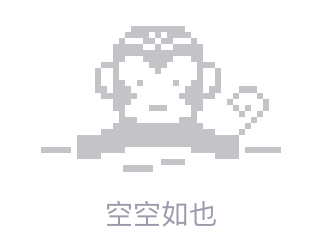# 在PHP中通过键删除JSON子项

My goal is to have a function that can remove a specified json child, which could also be nested inside deeper.

My function looks like this:

``````private function removeJsonChild(String \$jsonKey, String \$jsonString)
{
\$json = json_decode(\$jsonString, true);
\$arr_index = array();

foreach (\$json as \$key => \$value) {
if (is_array(\$value)) {
\$json[\$key] = \$this->removeJsonChild(\$jsonKey, json_encode(\$value));
}

if (\$key == \$jsonKey) {
\$arr_index[] = \$key;
}
}

foreach (\$arr_index as \$i) {
unset(\$json[\$i]);
}

return json_encode(\$json);
}
``````

The function would work if i wouldn't check if a `\$value` is an array and then call the function again recursively. But there is the problem i think. In the statement where i assign the return value of the function to `\$json[\$key]`. What am i doing wrong?

EDIT: definitely forgot a `json_decode`. New code looks like this:

``````private function removeJsonChild(String \$jsonKey, String \$jsonString)
{
\$json = json_decode(\$jsonString, true);
\$arr_index = array();

foreach (\$json as \$key => \$value) {
if (is_array(\$value)) {
\$json[\$key] = json_decode(\$this->removeJsonChild(\$jsonKey, json_encode(\$value)));
}

if (\$key == \$jsonKey) {
\$arr_index[] = \$key;
}
}

foreach (\$arr_index as \$i) {
unset(\$json[\$i]);
}

return json_encode(\$json);
}
``````

EDIT2:

The function works now, however it slightly changes the json schema.

An JSON like this:

``````[
{
"id": 1,
}
]
``````

now becomes this:

``````{
"1": {
"id": 1,
}
}
``````2019/05/23 07:27
• json
• php
• 点赞
• 收藏
• 回答
私信

#### 1个回复